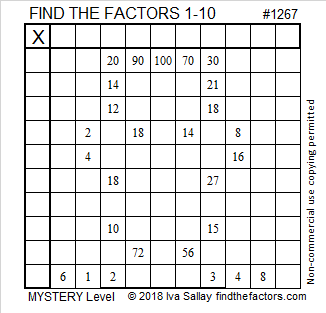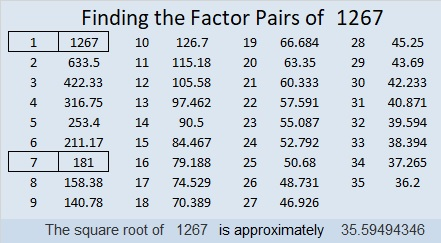# 1267 Frankenstein Mystery

There are legends of Dr. Frankenstein creating a monster years ago. Nowadays Frankenstein’s Monster can often be seen walking through neighborhoods on Halloween night. This puzzle looks a little bit like him.Print the puzzles or type the solution in this excel file: 10-factors-1259-1270

But if you take all the color away, he looks completely different and quite harmless:Now I’ll share some information about the number 1267:

• 1267 is a composite number.
• Prime factorization: 1267 = 7 × 181
• The exponents in the prime factorization are 1 and 1. Adding one to each and multiplying we get (1 + 1)(1 + 1) = 2 × 2 = 4. Therefore 1267 has exactly 4 factors.
• Factors of 1267: 1, 7, 181, 1267
• Factor pairs: 1267 = 1 × 1267 or 7 × 181
• 1267 has no square factors that allow its square root to be simplified. √1267 ≈ 35.594941267 is the sum of nine consecutive prime numbers:
113 + 127 + 131 + 137 + 139 + 149 + 151 + 157 + 163 = 1267

1267 is the hypotenuse of a Pythagorean triple:
133-1260-1267 which is 7 times (19-180-181)

This site uses Akismet to reduce spam. Learn how your comment data is processed.